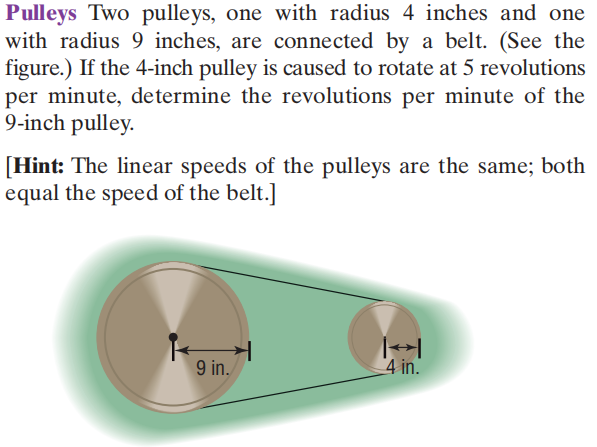### Still have math questions?

Algebra
QuestionPulleys Two pulleys, one with radius $$4$$ inches and one with radius $$9$$ inches, are connected by a belt. (See the figure.) If the $$4$$ -inch pulley is caused to rotate at $$5$$ revolutions per minute, determine the revolutions per minute of the

$$9$$ -inch pulley. [Hint: The linear speeds of the pulleys are the same; both equal the speed of the belt.]